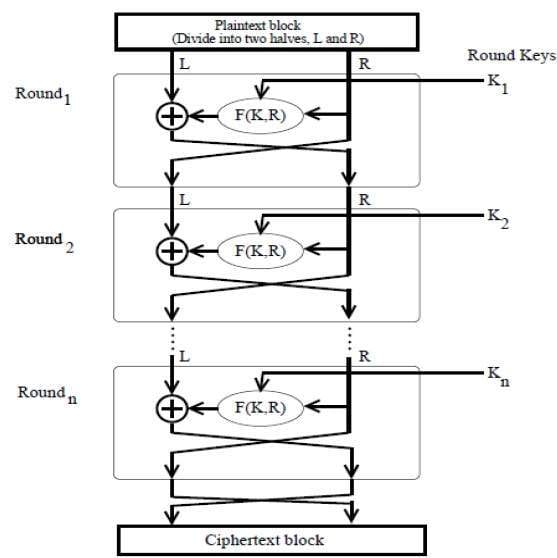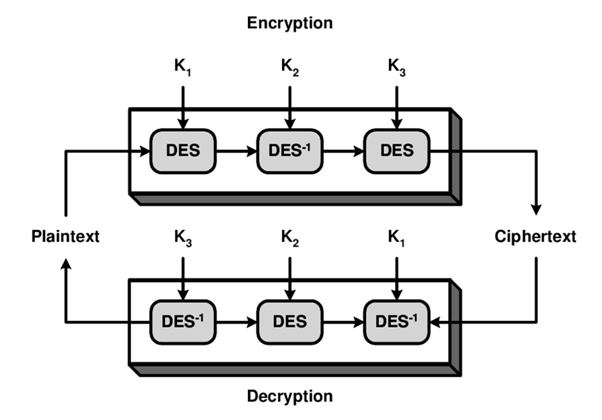LEARN

# The Triple DES Intro: Triple Data Encryption Standard

Triple Data Encryption Standard (Triple DES) is a symmetric block cipher-based cryptography standard that uses fixed length keys with three passes of the DES algorithm. As a symmetric cryptographic scheme, DES implementations rely on the same secret keys shared between the sender and the recipient.

As we’ll see later, Triple DES was developed as a way to prevent man in the middle attacks. Let’s take a look at Triple DES — and we’ll start first with DES.## Origin story: Data encryption standard

Encryption keys need to be protected against a confidentiality, integrity or availability (CIA) compromise common in a Man in the Middle attack. As such, multiple rounds of encryption and decryption rounds are implemented with the Data Encryption Standard (DES) algorithm.

In order to reduce the dependence on memory, DES offers an online approach to cryptographic implementation: applying encryption and decryption on the fly, such that the keys are not required to be stored during the transmission.

DES is a block cipher-based scheme, which refers to the memoryless algorithmic implementation to transform large plaintext data units into blocks of cipher text for encryption — and vice versa for decryption. This process is repeated multiple times for pairwise generation of plaintext-ciphertext blocks.

Developed in 1977, the Data Encryption Standard has been the world de facto choice for block cipher-based cryptosystem implementations between 1980 to 2004. Specifically, DES is based on the Feistel cipher design model that uses the substitution and permutation operations alternatively as shown here:(Image source)

The Feistel block uses multiple rounds functions between a data block and a subkey, with output returning a block of the same size as the input. The first half of the input block is encrypted with the subkey, and the output undergoes an XOR function with the remaining half of the data block. By repeating this process multiple times, the data is encrypted and the mathematical functions are invertible. This means that the same block process or the key schedule can be inverted to decrypt a ciphertext.

### DES operations

Let’s take a look at the DES cryptography operations:

1. Expansion: The half block of 32 bits is expanded to length of 48 using expansion permutations.
2. Key mixing: An XOR operation is applied to this data with the 16 subkeys of 48-bit length for each round.
3. Substitution: The resultant block is further divided into smaller blocks of 6 bits and processed with the Substitution box, which generates a nonlinear transformation of the input data using a lookup table for the S-box.
4. Permutation: The output is rearranged using a fixed permutation using the P-box.

## Triple DES algorithm

Now, let’s turn to Triple DES. Here is a description of the Triple-DES algorithm implementation:(Image source)

The Encryption scheme can be denoted as:

• C(x) = EK3(DK2(EK1(P(x))))
• Encrypt plaintext using the key K1; decrypt using key K2 and encrypt the resultant using K3.

And the Decryption scheme can be denoted as:

• P(x) = DK3(EK2(DK1(C(x))))
• Decrypt the plaintext using the key K1; encrypt using key K2 and decrypt the resultant using K3.

Where:

• P(x) is the plaintext
• C(x) is the ciphertext
• EK is the encryption using a key K
• DK is the decryption using a key K

## When to use DES vs Triple DES

The DES block cipher method is particularly suitable for low-memory hardware processing units — the same code and circuit implementation can be used for both the encryption and decryption process.

The Triple-DES scheme was introduced in 1978, only a year later. It uses three DES iterations as the encryption and decryption process. This scheme uses a 168-bit key, offers improved security — but is slower than the standard DES implementation.

In 1999, a variation of Triple DES was introduced which uses the same keys for all three passes of the DES (and inverse-DES) algorithm. This is known as the 2-TDES algorithm and uses a key length of 112 bits.The idea behind using three DES passes instead of two is designed to prevent Man in the Middle attacks. In a setting with Double-DES implementation, an adversary could read the encrypted output of the DES passes at each end of the stream. They could then use a brute-force attack to find relationships between the block set and the mathematical operator. Keys that produce the same result in either direction could also be identified as possible solutions to the DES implementation. This is known as the classical collision attack — it’s possibly faster than using a brute-force attack.

According to NIST (publication 800-67), here are the specs:

• Single DES offers 56 bit security which was already inadequate compared to alternative schemes in 1977.
• Double DES improved the security to 72-bit level.
• The 2-key Triple DES which uses the same key values for the Keys K1 and K3 offer an 80-bit security level, which has been acceptable until 2009.
• Finally, the Triple DES scheme that uses three different keys offers a 100-bit security level which is considered acceptable until the year 2030.

## Other cryptographic algorithms

An alternative is the AES algorithm that is as strong as Triple DES — but more efficient with its 128 bit block length and key length of 128-192-256 bits. You’ll also want to consider post-quantum cryptography and overall key management.

## What is Splunk?

This posting does not necessarily represent Splunk's position, strategies or opinion.Posted by